﻿ Slope of the v vs. t Graph, Acceleration | Zona Land Education

# Slope of the v vs. t Graph, Acceleration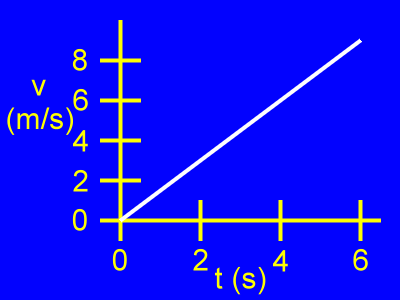Here is a v vs. t, or velocity vs. time, graph.

Velocity (v) is vertical.

Time (t) is horizontal.

Initially, when t = 0 s, the velocity of the object is v = 0 m/s.

From then on as time passes the object moves with a greater and greater positive velocity.

Let's look at two points on this graph....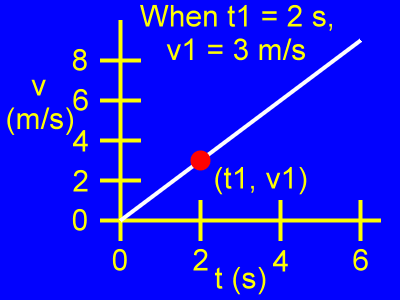Examine the first point, (t1, v1).

When t1 = 2 s, then v1 = 3 m/s.

Examine the second point, (t2, v2).

When t2 = 6 s, then v2 = 9 m/s.

Let's find the slope of this graph using those two points...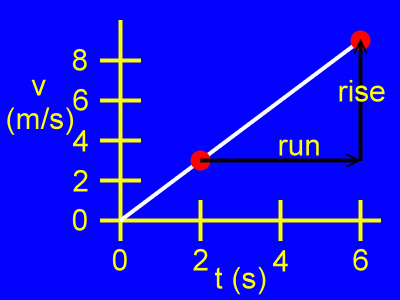Using those two points, here is the rise and the run of the slope of this v vs. t graph.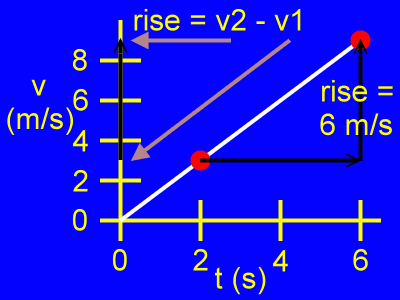Here, the rise is the difference of the velocity coordinates, or v2 - v1, as in:

rise = v2 - v1

rise = 9 m/s - 3 m/s

rise = 6 m/s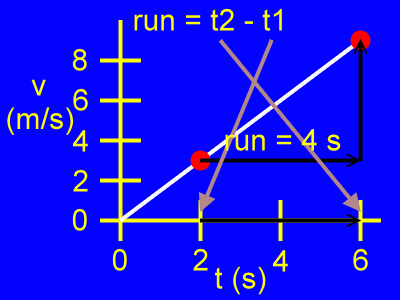Here, the run is the difference of the time coordinates, or t2 - t1, as in:

run = t2 - t1

run = 6 s - 2 s

rise = 4 s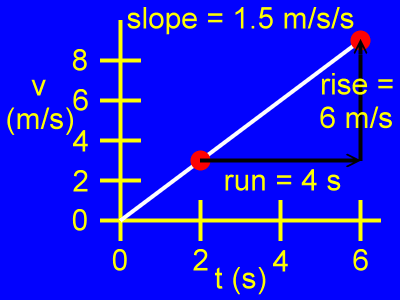The slope of this graph is a change in velocity divided by a change in time, as in:

slope = rise / run

slope = 6 m/s / 4 s

slope = 1.5 m/s/s (or m/s2)

This slope is the acceleration of the object, since acceleration is defined as the change in velocity divided by the change in time.

So, acceleration = 1.5 m/s/s.

Custom Search